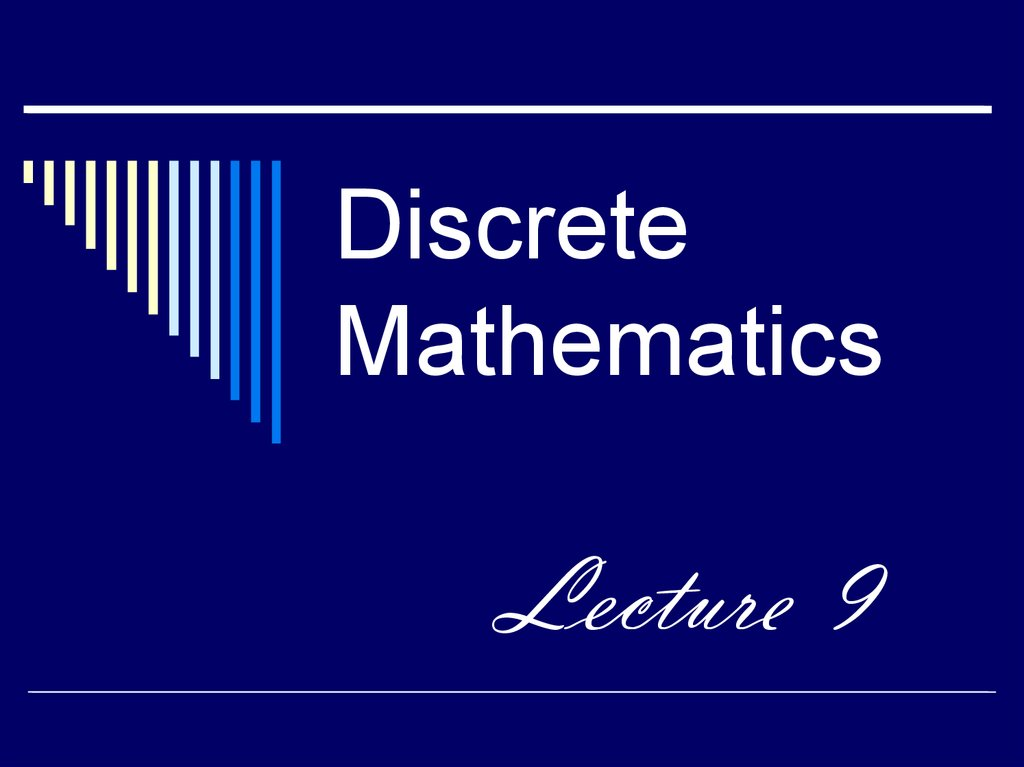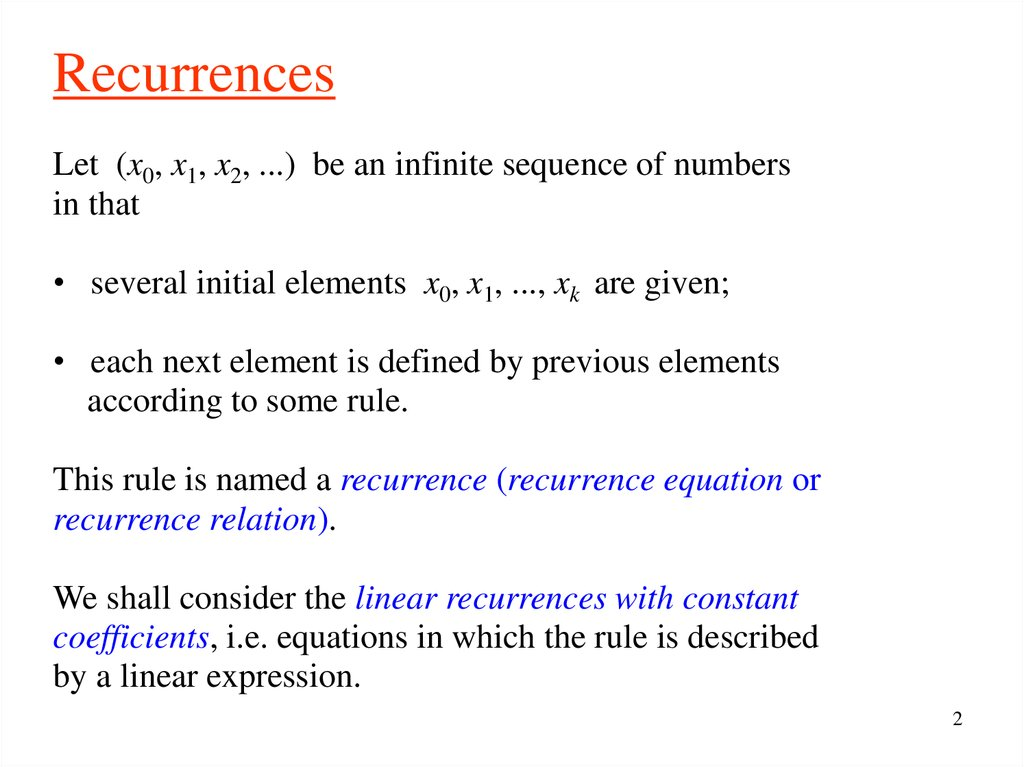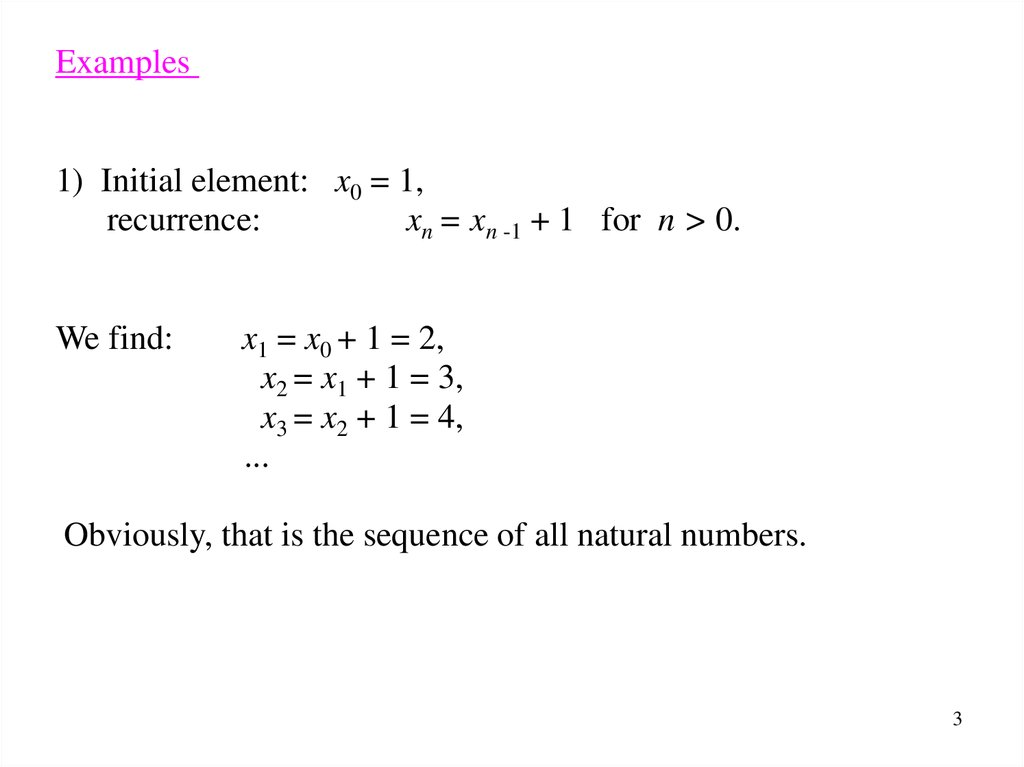# Discrete mathematics

Lecture 9

## 2.

Recurrences
Let (x0, x1, x2, ...) be an infinite sequence of numbers
in that
• several initial elements x0, x1, ..., xk are given;
• each next element is defined by previous elements
according to some rule.
This rule is named a recurrence (recurrence equation or
recurrence relation).
We shall consider the linear recurrences with constant
coefficients, i.e. equations in which the rule is described
by a linear expression.
2

## 3.

Examples
1) Initial element: x0 = 1,
recurrence:
xn = xn -1 + 1 for n > 0.
We find:
x1 = x0 + 1 = 2,
x2 = x1 + 1 = 3,
x3 = x2 + 1 = 4,
...
Obviously, that is the sequence of all natural numbers.
3

## 4.

2) Initial element: x0 = 1,
recurrence:
xn = 2xn -1
We find:
for n > 0.
x1 = 2x0 = 2,
x2 = 2x1 = 4,
x3 = 2x2 = 8,
...
Obviously, that is the sequence of degrees of 2:
x n 2 n.
4

## 5.

First order linear recurrences
The general linear recurrence of the first order has the form
x n ax n 1 b,
where a and b are given constants, n > 0.
If an initial element x0 is also given then we can compute
sequentially the other elements:
x1 ax0 b,
x 2 ax1 b a (ax0 b) b a 2 x0 ab b,
...
5

## 6.

Any element xn, n > 0, is uniquely defined by a, b, and x0.
Can we write a general formula for it?
First we shall consider the following two special cases:
1. a = 1.
2. b = 0.
6

## 7.

1. a = 1.
The equation has the form
x n x n 1 b.
We can find
x1 x0 b,
x 2 x1 b x0 2b,
x3 x 2 b x0 3b,
...
Obviously,
x n x0 nb
for any n.
(It can be easy proved by induction on n).
This sequence is an arithmetic progression.
7

## 8.

2. b = 0.
The equation has the form
x n ax n 1 .
We can find
x1 ax0 ,
x 2 ax1 a 2 x0 ,
x3 ax 2 a x0 ,
3
...
Obviously,
x n a n x0
for any n.
This sequence is a geometric progression.
8

## 9.

Now we consider the general case
x n ax n 1 b.
(1)
First we shall reduce the equation to a simplified form with
a help of the change of unknown
x n y n s,
(2)
where yn is a new unknown, s is a constant which value
we shall determine later.
Substituting of (2) in (1) we get
y n s a ( y n 1 s ) b,
or
9

## 10.

y n ay n 1 as b s.
Let us select such s that
as b s 0,
i.e.
b
s
.
1 a
Note that for a = 1 this expression is not defined.
But the case a = 1 had been considered previously.
Further we suppose that a 1.
Then we obtain the equation
y n ay n 1 .
10

## 11.

This equation has a simplest form (case 2), its solution is
yn a n y0 .
Because of
xn y n s
we obtain
x n s a ( x0 s)
n
and it remains to substitute the expression for s:
b b
x n a x0
.
1 a 1 a
n
11

## 12.

We don't recommend you to remember this formula. It is rather
the solution method. It includes the following three stages.
1. Reduction of equation to the simplest form by the change of
unknown xn = yn + s and choice of suitable value for s.
2. Solving of the obtained simplest equation.
12

## 13.

Note that the solution has the form
x n c1 a n c 2
where c1 and c2 are some constants.
We see that the dependence of xn on n is expressed
as an exponential function.
13

## 14.

Example:
Towers of Hanoi
The French mathematician Edouard Lucas invents in 1883
the following problem. Eight disks of different sizes are
threaded on one of three pegs in order of size decreasing.
Goal is to transpose the disks in the same order onto another
peg. It is allowed to move only one disk at a time and it is
forbidden to put a larger disk on the smaller one. How many
steps are needed for this? (A step is a movement of a disk)
14

## 15.

Let us consider this problem in a general form when there
are n disks.
Let Tn be the minimum number of steps needed to move
n disks from one peg to another.
Obviously, T1 = 1.
It is easy to see that T2 = 3:
15

## 16.

We can transpose three disks in the following manner:
1) move two disks as above (3 steps)
2) move the largest disk (1 step)
3) move two disks again (3 steps)
Thus T3 = 3 + 1 + 3 = 7.
16

## 17.

In general case we must transpose n – 1 smaller disks
before we move the largest disk.
It requires Tn -1 steps.
After moving the largest disk it is necessary again to
transpose n – 1 smaller disks.
It requires also Tn -1 steps.
Hence we have the recurrence
Tn 2Tn 1 1, n 1.
If we let
T0 0
then the equality is also valid for n = 1.
17

## 18.

We solve this equation in three described stage.
1. Reduction.
Let
then
Tn Yn s
Yn s 2Yn 1 2 s 1.
Take s = 1 and get the equation
Yn 2 n Y0 .
2. Solving of simplified equation.
Yn 2Yn 1 .
Yn Tn 1,
Y0 T0 1 1.
Tn 2 n 1.
18

## 19.

Second order linear recurrences
General linear recurrence equation of second order has the form
x n ax n 1 bx n 2 c.
where a, b, and c are given constants, n > 1.
We shall consider first the homogeneous equation (c = 0):
x n ax n 1 bx n 2 .
(3)
19

## 20.

If we know the two initial elements x0 and x1 then
we are able to compute sequentially the other elements:
x 2 ax1 bx0 ,
x3 ax 2 bx1 a (ax1 bx0 ) bx1 (a 2 b) x1 abx0
and so on.
Every element xn, n > 1, is uniquely defined by a, b, x0, x1.
We also can obtain a general formula for xn in this case .
20

## 21.

We shall look for a solution in the exponential form:
xn n
where is unknown constant (the idea goes from the
solutions form of the first order recurrence).
Substitution of this expression in the equation (3) gives
a
n
n 1
b
n 2
.
n 2 and we get
It may be reduced by
a b.
2
21

## 22.

Thus must be a root of the equation
2 a b 0
called a characteristic equation.
There are the two possibilities.
A. The characteristic equation has two distinct roots
1 and 2 .
B. There is one root 1 = 2.
(the discriminant is zero: a2 + 4b = 0).
22

## 23.

A. The characteristic equation has two distinct roots
1 and 2.
Then both sequences
x n 1n
and
x n 2n
satisfy the equation (3).
But we need solution with given x0, x1.
Can we achieve it?
23

## 24.

The homogeneous linear equation has two following
important properties:
1) if a sequence
x0 , x1 , , x n ,
satisfies the equation (3) and a is some constant then
the sequence
ax0 , ax1 , , ax n ,
also satisfies this equation
(to verify this statement it is sufficient to substitute axn in the
24

## 25.

2) if sequences
x0 , x1 , , x n ,
and
x0 , x1 , , x n ,
both satisfy the equation (3) then the sequence
x0 x0 , x1 x1 , , x n x n ,
also satisfies this equation.
(If we substitute
then we get
x n x n in the equation instead of xn
x n x n ax n 1 ax n 1 bx n 2 bx n 2
and it is fulfilled since
and
x n ax n 1 bx n 2
x n ax n 1 bx n 2 )
25

## 26.

Due to properties 1), 2) we may affirm that the sequence
c1 1n c 2 2n
satisfies the equation (3) for any constants c1, c2. This
sequence is called a general solution of an equation (3).
Can we fit c1, c2 in such a way that two initial elements of the
sequence would be x0 and x1? We shall try:
n 0
x0 c1 10 c 2 20 c1 c 2 ,
n 1
x1 c1 11 c 2 21 c1 1 c 2 2 .
26

## 27.

Thus we get a system of two linear equations with two
unknowns c1, c2:
c1 c 2 x0 ,
c1 1 c 2 2 x1 .
The determinant of this system is
1
1
1 2
2 1 0
as
1 2 .
Hence there exists a unique solution c1, c2
and we obtain a solution of equation (3) with given
start-up values.
27

## 28.

B. The characteristic equation has a single root .
In this case the general solution has the form
x n (c1 c 2 n)
n
(without a proof).
Constants c1 and c2 may be found using the initial
values:
n 0
x0 c1 ,
n 1
x1 (c1 c 2 ) .
28

## 29.

So we have the following algorithm for solving of a linear
homogeneous recurrence equation of the second order.
1. Write the characteristic equation and solve it.
2a. If the characteristic equation has two distinct roots
1 and 2 then write the general solution in the form
x n c1 1n c 2 2n .
2b. If the characteristic equation has a unique root
then write the general solution in the form
x n (c1 c 2 n) n .
3. Write equation system for c1, c2 using given initial
values x0, x1 and solve it.
4. Substitute the found values of constants
in the general solution.
29

## 30.

Examples
1)
x n 2 x n 1 3 x n 2 ,
x0 1, x1 2.
2 2 3 0.
The characteristic equation:
Roots:
1 3, 2 1.
The general solution:
Equations for c1, c2:
c1 3 n c 2 ( 1) n .
c1 c 2 1,
3c1 c 2 2.
3
1
c1 , c 2
4
4
Solution of the equation:
n 1
n
3 n 1
3
(
1
)
x n 3 ( 1) n
.
4
4
4
30

## 31.

Examples
2)
x n 4 x n 1 4 x n 2 ,
The characteristic equation:
Unique root:
x0 1, x1 4.
2 4 4 0.
2.
The general solution:
Equations for c1, c2:
(c1 c 2 n)2 n.
c1 1,
2c1 2c 2 4.
c1 1, c 2 1
Solution of the equation :
xn (1 n) 2 n.
31

## 32.

Inhomogeneous equation
An inhomogeneous linear recurrence of the second order
x n ax n 1 bx n 2 c
may be reduced to homogeneous equation in the
same way as in the case of recurrences of the first order.
We introduce a new unknown yn
xn y n s
and select such s that the constant term vanishes:
c
s
.
1 a b
32

## 33.

If a + b 1 then s exist and we obtain a homogeneous
If a + b = 1 then s does not exist. In this case one has to
make the other change of unknown:
x n y n sn
and again select such s that equation becomes
homogeneous.
33

## 34.

Fibonacci numbers
Leonardo Fibonacci (1170 – 1250) also known as
Leonardo Pisano, was an Italian mathematician.
He is the best known due to the discovery of the Fibonacci
numbers and because of his role in the introduction of the
modern Arabic decimal system for writing numbers in
Europe.
34

## 35.

The Fibonacci numbers are elements of the sequence
0, 1, 1, 2, 3, 5, 8, 13, 21, 34, ... .
In this sequence each element beginning with the third is
equal to the sum of two preceding elements:
1 = 1 + 0,
2 = 1 + 1,
3 = 2 + 1,
5 = 3 + 2,
...
35

## 36.

If we denote the n-th element of the sequence by
Fn (n = 0, 1, 2, ... ) then the rule may be written as
Fn Fn 1 Fn 2 .
It is a linear recurrence equation of the second order.
There are also initial values
F0 0, F1 1
and we can find a general formula for the Fibonacci
number.
36

## 37.

The characteristic equation for this recurrence is
2 1 0.
It has two roots
1
1 5
,
2
2
1 5
.
2
The general solution of the recurrence is
n
n
1 5
1 5
c2
.
c1
2
2
37

## 38.

Now we use the initial values for writing the equations
for the constants c1 and c2:
c1 c 2 0,
c1 1 c 2 2 1.
Solving this system we find
1
1
c1
,
1 2
5
c2
1
5
,
and obtain the formula for the Fibonacci numbers:
n
n
1 1 5
1 1 5
.
Fn
5 2
5 2
38

## 39.

Example:
Sparse words
A binary word containing no two consecutive 1’s will be called
a sparse word.
For example, the word
is sparse
while words
are not sparse.
0100101000101
0101100011 and 0011110
Denote the number of all sparse words of length
n
by Un.
39

For small
n
n
we have:
0
1
2
3
4
λ
0
1
00
01
10
000
001
010
100
101
0000
0001
0010
0100
0101
1000
1001
1010
3
5
8
Sparse
words
Un
1
2
40

## 41.

What is U5 ?
If is a sparse word of length 5 and the first letter of
is 0 then
= 0β
where β is a sparse word of length 4.
There are 8 of such words:
00000
00001
00010
00100
00101
01000
01001
01010
41

## 42.

If the first letter in is 1 then the second letter must be 0
and
= 10γ
where γ is any sparse word of length 3.
There are 5 of such words:
10000
10001
10010
10100
10101
At all we have
U 5 U 4 U 3 8 5 13.
42

## 43.

In general case let be a sparse word of the length
n.
• If the first letter of is 0 then = 0β where β is
any sparse word of length n
1.
There are Un -1 such words.
• If the first letter in is 1 then the second letter must be 0
and = 10γ where γ is any sparse word of length
n 2.
There are Un -2 such words.
43

## 44.

Thus we obtain the recurrence for these numbers:
U n U n 1 U n 2
for n ≥ 2.
It is the same recurrence as for Fibonacci numbers.
However the start-up values are other:
U0 = 1, U1 = 2.
But we see that U0 and U1 coincide with two successive
Fibonacci numbers:
U 0 F2 , U 1 F3 .
Consequently,
U n Fn 2
for n = 0, 1, 2, ... .
44